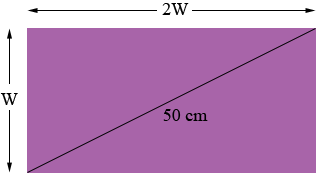SEARCH HOMEMath Central Quandaries & QueriesQuestion from Vickie, a student: A rectangle is 2 times longer than it is wide. It has a diagonal length of 50 centimeters. Find the length and the width of the rectangle. Round your answers to the nearest tenth. Show your work.Hi Vickie,

Draw a diagram! If the width is W then the length must be 2W.This looks like a job for Pythagoras.

PennyMath Central is supported by the University of Regina and The Pacific Institute for the Mathematical Sciences.